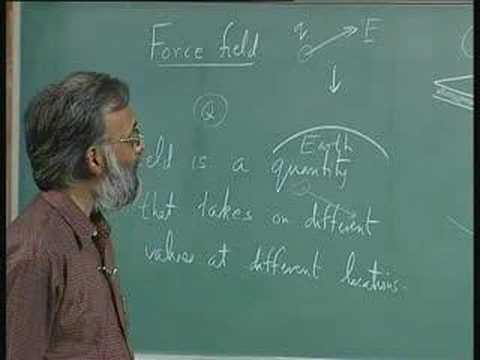NPTEL Courses

# Electromagnetic Fields

This is a course on electromagnetic theory, aimed at EEE students who are doing power electronics, machines, power systems and other subjects. The course consists of four main areas: electrostatics, magnetostatics, time dependent fields and waves. Electrostatics introduces the electric field, electric potential and an important equation namely Poisson's equation. The second part has to do with the magnetic field, and we will study how currents produce magnetic fields. The third part deals with Faraday's law. This is the crucial part of the course for EEE students, because it is a combination of Ampere's law and Faraday's law that makes all electric machines, generators, and almost all power generation systems to work. At the last part of the course, we will introduce Maxwell's equations and waves. (from nptel.ac.in )

Instructor

Electronics
2007

#### Lecture 01 - Course Introduction, Introduction to Vector